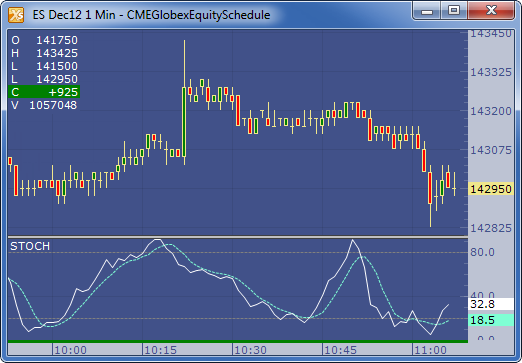← Back to X_TRADER® Help Library

### X_STUDY® Documentation

#### Stochastic (STOCH)

##### Description

The Stochastic (Stoch) normalizes price as a percentage between 0 and 100. Normally two lines are plotted, the %K line and a moving average of the %K which is called %D. A slow stochastic can be created by initially smoothing the %K line with a moving average before it is displayed. The length of this smoothing is set in the Slow K Period. Without the initial smoothing ( i.e., setting the Slow K Period to a value of 1 ) the %K becomes the ‘Raw %K’ value, and is also known as a fast stochastic.

##### Formula

Fast %K = 100 SMA ( ( ( Close - Low ) / ( High - Low ) ),Time Period )

Slow %K = SMA ( Fast  %K, Kma )

Slow %D = SMA ( Slow K%, Dma )

Where:

• Close = the current closing price
• Low = the lowest low in the past  n periods
• High = the highest high in the past n periods
• Kma = Period of Moving Average used to smooth the  Fast %K Values
• Dma = Period of Moving Average used to smooth the Slow %K Values
##### Example